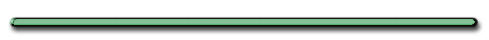Home"The most beautiful thing
we can experience
is the mysterious...
It is the source
of all true art
and all science."

- Einstein

Unit 1 - FORCES

Forces - any straight-line push or pull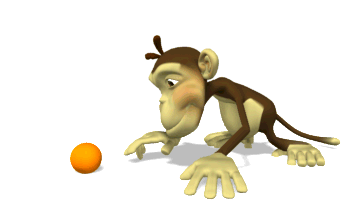A. Representing Forces

1. Units of force

a)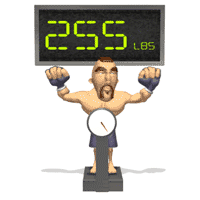lb. (English)

b) Newton (metric) (weight of 1 apple)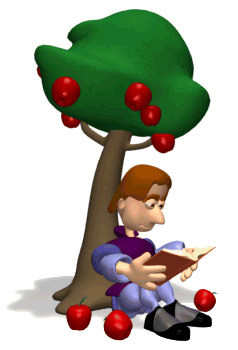2. Forces are VECTORS

- described using:

- magnitude (size)

- directionReview - Scalar (magnitude only)

ex) time, distance

Solving problems with vectors require
a special branch of mathematics

3. When solving problems and drawing pictures, forces are represented as arrows drawn to some selected scale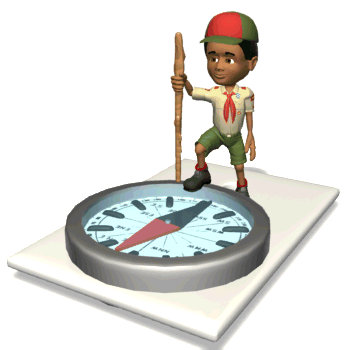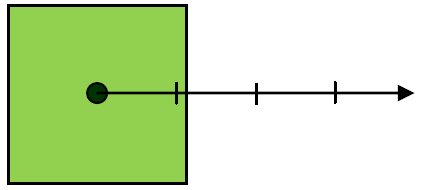Scale:  1 cm = 10 N

F = 40 N East

*Length shows magnitude (size)

**arrow shows directionCombining Forces